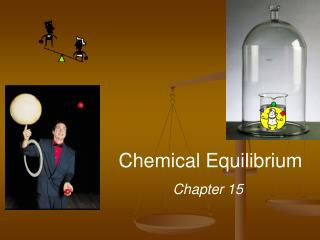DownloadDownload PresentationChemical Equilibrium

# Chemical Equilibrium

Télécharger la présentation## Chemical Equilibrium

- - - - - - - - - - - - - - - - - - - - - - - - - - - E N D - - - - - - - - - - - - - - - - - - - - - - - - - - -
##### Presentation Transcript

1. Chemical Equilibrium Chapter 15

2. H2O (l) H2O (g) N2O4(g) 2NO2(g) Equilibrium - state in which there are no observable changes with time • Achieved when: • rates of the forward and reverse reactions are equal and • concentrations of the reactants and products remain constant • dynamic equilibrium Physical equilibrium Chemical equilibrium colorless red-brown

3. Fig 15.1 The N2O4(g) ⇌ 2 NO2 (g) equilibrium colorless red-brown

4. Since both reactions are elementary, we use kinetics, • Forward reaction: N2O4 (g) 2 NO2 (g) • Rate Law: Rate = kf [N2O4] • Reverse reaction: 2 NO2 (g) N2O4 (g) • Rate law: Rate = kr [NO2]2 • At equilibrium: Ratef = Rater or kf [N2O4] = kr [NO2]2

5. N2O4(g) 2NO2(g) Fig 15.2 (a) Achieving chemical equilibrium for: Start with N2O4 Equilibrium occurs when concentrations no longer change

6. N2O4(g) 2NO2(g) Fig 15.2 (b) Achieving chemical equilibrium for: Equilibrium occurs when kf and kr are equal

7. Equilibrium Can Be Reached from Either Direction e.g., the Haber process to form ammonia N2 (g) + 3 H2 (g) ⇌ 2 NH3 (g) • Start with N2 and H2 or with NH3 • Result: same proportions of all three substances at equilibrium

8. aA + bB cC + dD [C]c[D]d KC = [A]a[B]b Equilibrium constant Law of Mass Action Must be caps! Lies to the right Lies to the left

9. constant N2O4(g) 2NO2(g) Table 15.1 Initial concentrations in gas phase at 100 oC Fig 15.1 Concentration changes approaching equilibrium

10. N2O4 (g) 2NO2 (g) Kc = Kp = In most cases Kc Kp P2 [NO2]2 NO2 [N2O4] P N2O4 The equilibrium constant may be expressed in terms of concentration or in terms of pressure Kp = Kc(RT)Δn Δn = moles of gaseous products – moles of gaseous reactants

11. N2 (g) + 3 H2 (g) 2 NH3 (g) Sample Exercise 15.2 p 634 In the synthesis of ammonia from nitrogen and hydrogen, Kc = 9.60 at 300 °C. Calculate Kpfor this reaction at this temperature

12. CO (g) + Cl2(g) COCl2(g) = 0.14 (0.012) · (0.054) [COCl2] [CO][Cl2] The equilibrium concentrations for the reaction between carbon monoxide and molecular chlorine to form COCl2 (g) at 74°C are [CO] = 0.012 M, [Cl2] = 0.054 M, and [COCl2] = 0.14 M. Calculate the equilibrium constants Kc and Kp. = 220 Kc= Kp = Kc(RT)Dn Dn = 1 – 2 = -1 R = 0.0821 (L·atm)/(mol·K) T = 273 + 74 = 347 K Kp= 220 ·[0.0821 · 347]-1 = 7.7